Courses

Alternating Current MSQ

10 Questions MCQ Test Topic wise Tests for IIT JAM Physics | Alternating Current MSQ

Description
Attempt Alternating Current MSQ | 10 questions in 45 minutes | Mock test for Physics preparation | Free important questions MCQ to study Topic wise Tests for IIT JAM Physics for Physics Exam | Download free PDF with solutions
*Multiple options can be correct
QUESTION: 1

A choke coil of resistance 5ω and inductance 0.6H is in series with a capacitance of 10µF. If a voltage of 200V is applied and the frequency is adjusted to resonance, the current and voltage across the inductance and capacitance are I0, V0 and V1 respectively. We have

Solution:

The frequency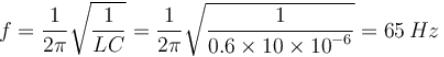The current at resonance is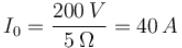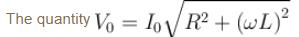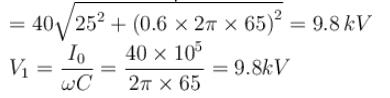The correct answers are: I0 = 40A , V0 = 9.8kVV1 = 9.8kV

*Multiple options can be correct
QUESTION: 2

An alternating emf of angular frequency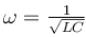is applied to a series LCR circuit. For this frequency of the applied emf, which of the following is not true.

Solution:

(ε = εsinωt)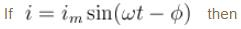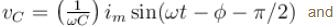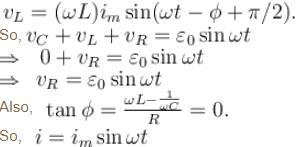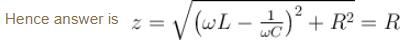As we know resonating angular  frequency is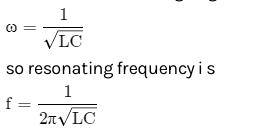so circuit is in resonance and impedence is made up of only resistance part

The correct answers are: The circuit is at resonance and its impedance is made up only of a reactive part, The sum of the potential differences across the inductance and capacitance equals the applied emf which is 180° ahead of phase of the current in the circuit, Impedance of the circuit is less than R

*Multiple options can be correct
QUESTION: 3

The value of current in two series LCR circuits at resonance is same when connected across a sinusoidal voltage source. Then which of the following is incorrect :

Solution: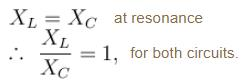The correct answers are: both circuits must be having same value of capacitance and inductor, in both circuits ratio of L and C will be same, both circuits must have same impedance at all frequencies.

*Multiple options can be correct
QUESTION: 4

Graph shows variation of source emf V and current i in a series RLC circuit, with time.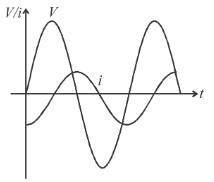Solution:

It is apparent from the graph that emf attains its maximum value before the current does, therefore current lags behind emf in the circuit. Nature of the circuit is inductive.
Value of power factor cos φ increases by either by decreasing L or by increasing C..

The correct answers are: The circuit is more inductive than capacitive., To increase the rate at which energy is transferred to the resistive load, L should be decreased.

*Multiple options can be correct
QUESTION: 5

In the circuit shown, resistance R = 100Ω, inductance L = 2H/π and capacitance  C = 8μF/π are connected in series with an ac source of 200volt and frequency f. If the readings of the hot wire voltmeters V1 and V2 are same then :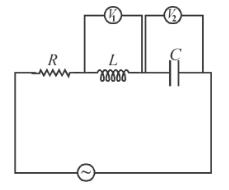Solution: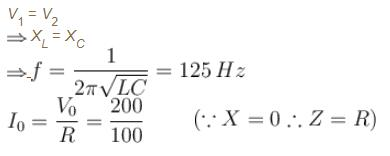= 2A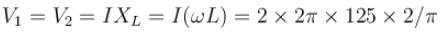= 1000 volt
The correct answers are: f = 125 Hz, current through R is 2AV1 = V2 = 1000 Volt

*Multiple options can be correct
QUESTION: 6

In an ac circuit shown below in figure the supply, voltage has a constant rms value V but variable frequency f. At resonance, the circuit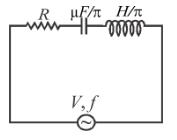Solution: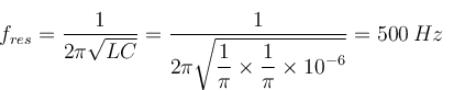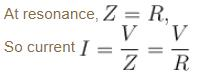When L and C are in series, voltage across capacitor and inductor is 180º out of phase.

The correct answers are: has current  given by I = V/R has a voltage across the capacitor which is 180° out of phase with that across the inductor

*Multiple options can be correct
QUESTION: 7

Resonance occurs in a series LCR circuit when the frequency of the applied emf is 1000Hz.

Solution:

(f = 900 Hz) < (fres = 1000 Hz), so XC > XL, circuit becomes capacitive and current leads emf.
At resistance, impedance in minimum.
Not only at resonance, but at all conditions voltage across L and C differs in phase by 180° when connected in series.

The correct answers are: when f = 900Hz, then the current through the voltage source will be ahead of emf of the source, the impedance of the circuit is minimum at f = 1000Hz

*Multiple options can be correct
QUESTION: 8

In the given ac circuit, which of the following is incorrect :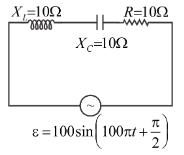Solution:

Since the circuit is at resonance so current in the circuit is in the phase with applied voltage. Voltage across inductro leads the current by π/2 and across a capacitor lag by π/2. So the voltage across resistance is lagging by 90 than the voltage across capacitor.

*Multiple options can be correct
QUESTION: 9

Which of the following statements is TRUE? Heat produced in a current carrying conductor depends upon :

Solution:

1) DC current will travel through the entirety of the wire -- AC current will tend to move on the outside of the conductor (see Skin Effect). This will cause slightly more ohmic heating in AC than in DC. That's why AC wire is usually stranded while DC wire is usually solid -- more strands = more surface area.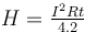the time for which the current flows in the conductor, the resistance of the conductor, the strength of the current.

*Multiple options can be correct
QUESTION: 10

In an RLC series circuit shown in figure the reading of voltmeters V1 and V2 are 100V and 120V, respectively. The source voltage is 130V. For this situation, mark out the correct statement(s).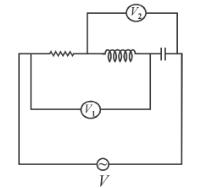Solution: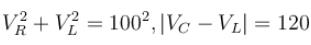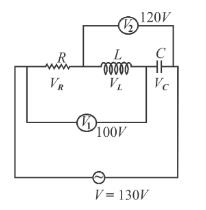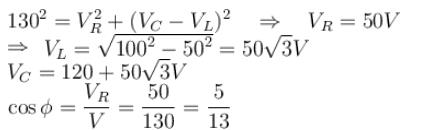Since VC < VL, so circuit is capacitive

The correct answers are: Voltage across resistor, inductor and capacitor are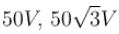and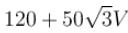respectively, Power factor of the circuit is 5/13,  The circuit is capacitive in natureUse Code STAYHOME200 and get INR 200 additional OFF Use Coupon Code

Track your progress, build streaks, highlight & save important lessons and more!

Similar ContentRelated tests